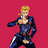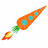V2EX = way to explore
V2EX 是一个关于分享和探索的地方

V2EX  ›  Vue.js

# 请问大神们， vuejs 从 vue.common.dev.js 换到 vue.common.js 后，为何网页出现问题

zhengfan2016 · 11 天前 · 784 次点击

``````mintimes: function(nS) {
m = parseInt(nS);
str = '';
if (m / 31536000 >= 1) {
str += toString(Math.floor(m / 31536000)) + "年";
m = m % 31536000;
}
if (m / 2592000 >= 1) {
str += toString(Math.floor(m / 2592000)) + "个月";
m = m % 2592000;
}
if (m / 86400 >= 1) {
str += toString(Math.floor(m / 86400)) + "天";
m = m % 86400;
}
if (m / 3600 >= 1) {
str += toString(Math.floor(m / 3600)) + "小时";
m = m % 3600;
}
if (m / 60 >= 1) {
str += toString(Math.floor(m / 60)) + "分钟";
m = m % 60;
}
str += toString(m) + "秒";
return str;
}
``````

5 条回复    2021-05-04 08:45:50 +08:001 zhengfan2016   11 天前 把所有的 tosiring(xxx)换成 xxx.tostring 就好了，emmmm 。2 whisky221   11 天前 时间最好还是都用 moment 或者 dayjs 吧，自己写的总归比不上被大量测试生产的3 suzic   11 天前 via Android 这个 tostring 是自己实现的方法吗4 Rocketer   10 天前 via iPhone @whisky221 同意，吃亏吃多了就老实了，我现在一些特简单的功能也要调包，有时花的时间比直接写还要多一点，但可靠更重要5 image72   10 天前 如果有支持 ts 的话 尽量加上 ts 类型, 或者你在前面判断下 "m" 在任何时候类型都为 number，以及默认数字值